Ex 4.1

Chapter 4 Class 8 Data Handling
Serial order wise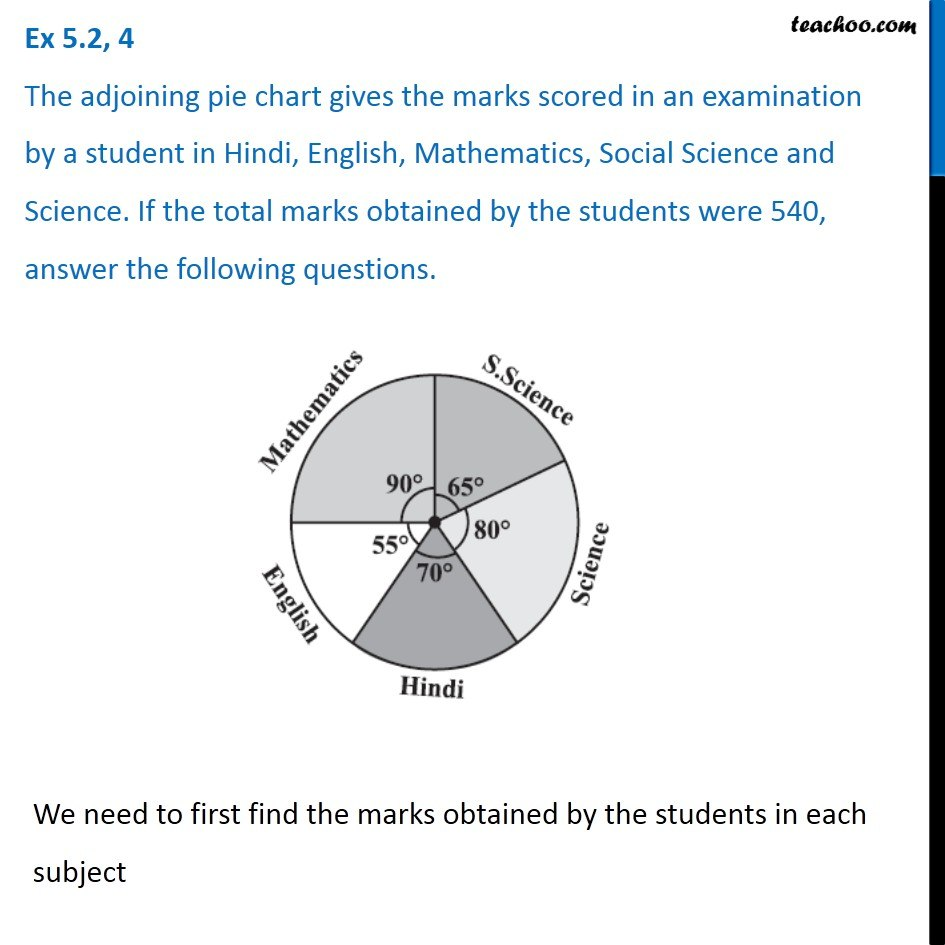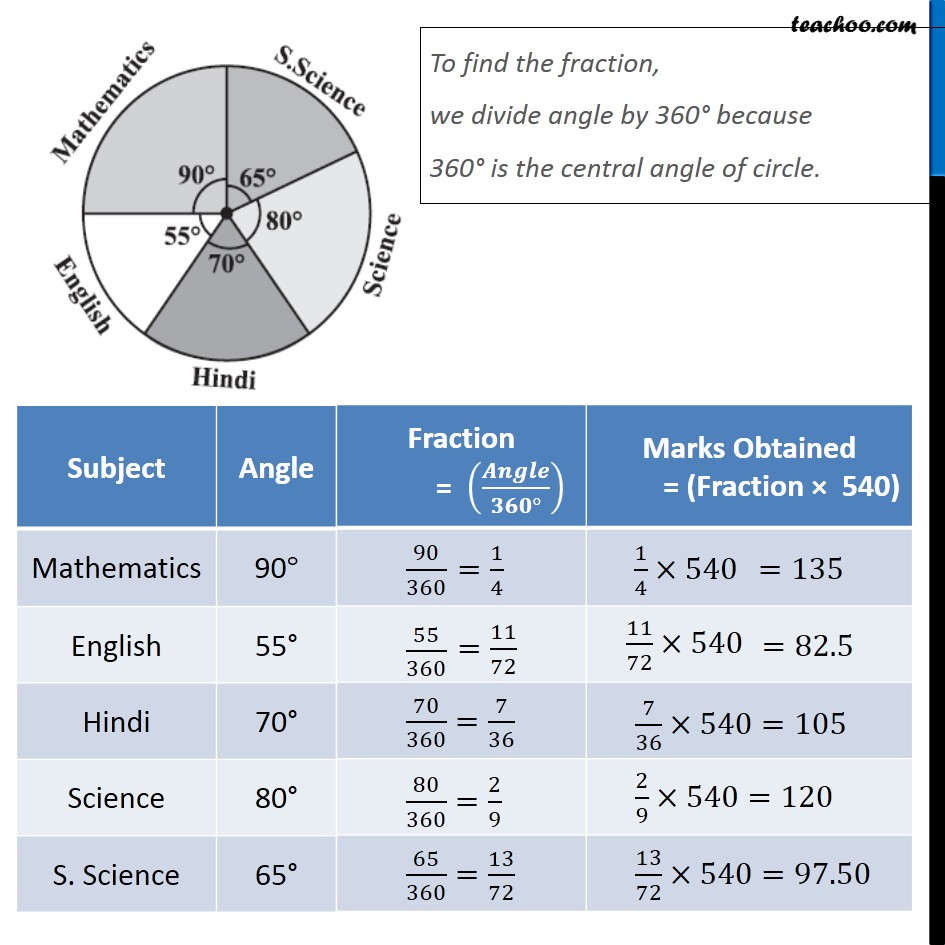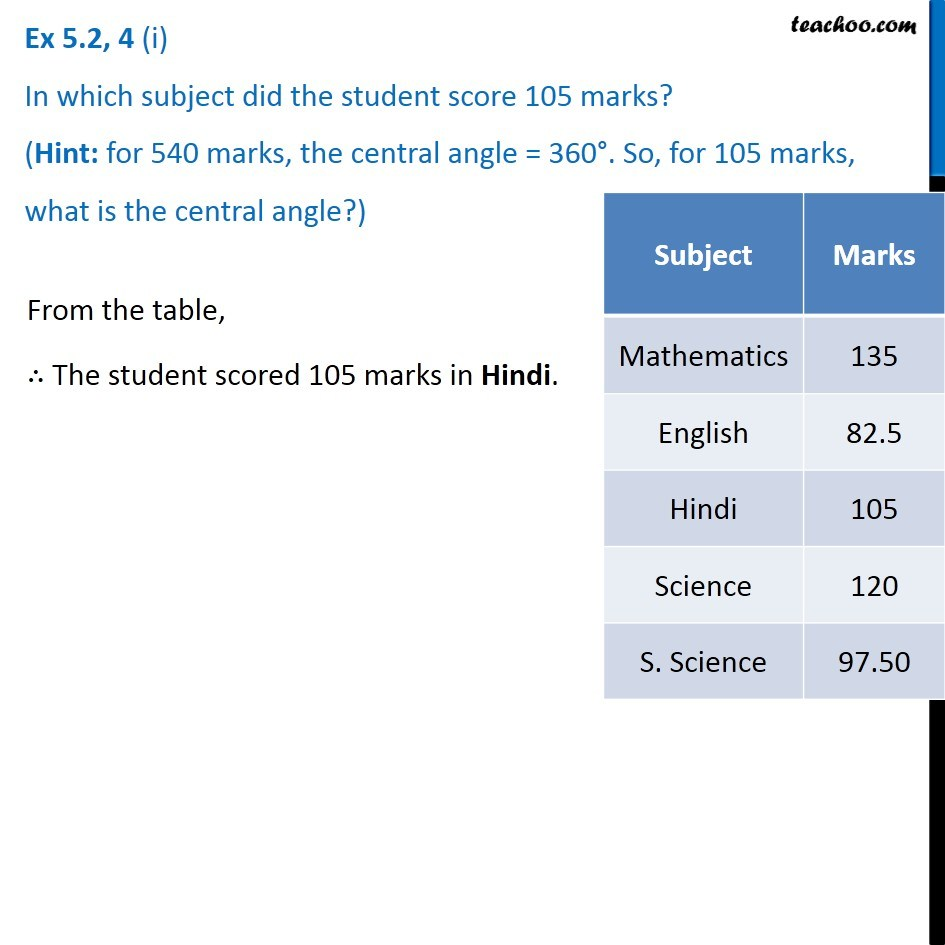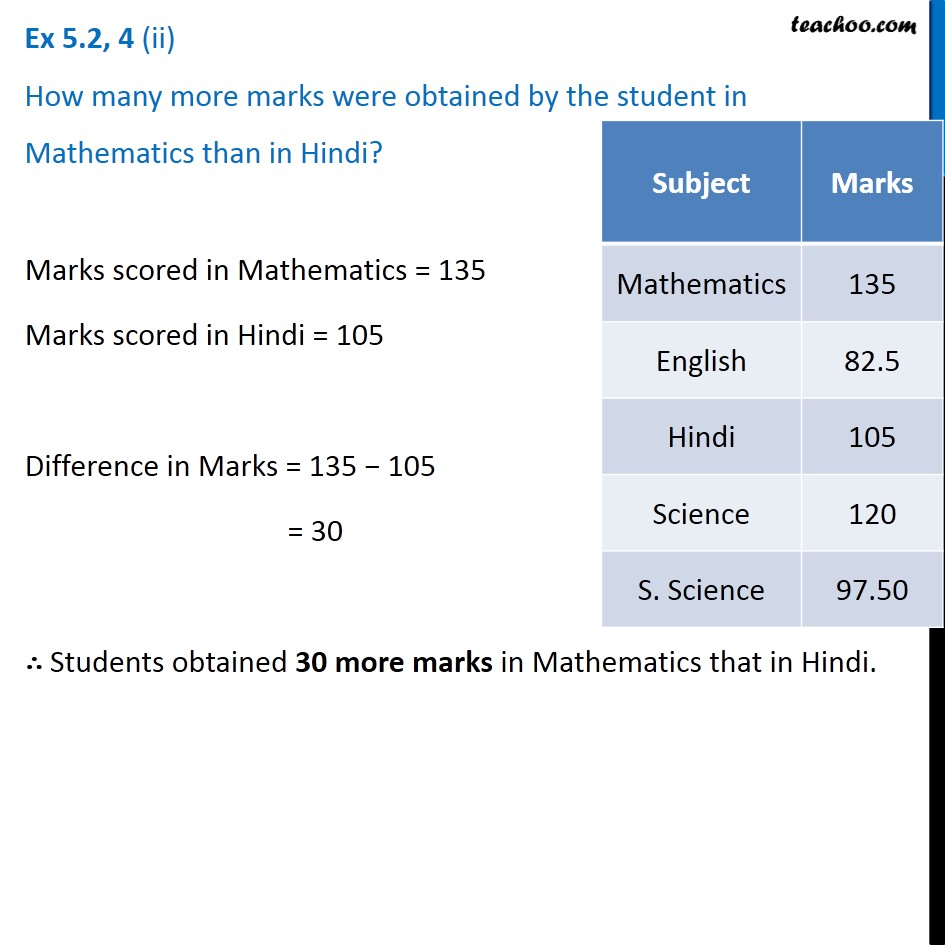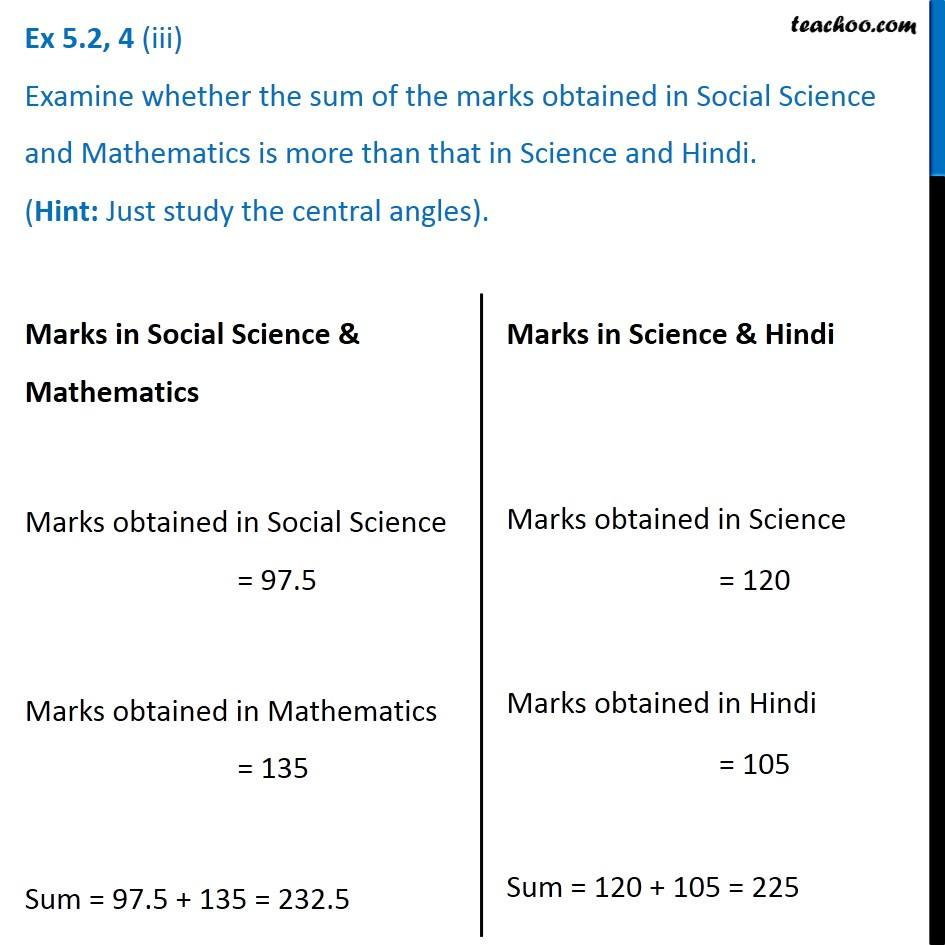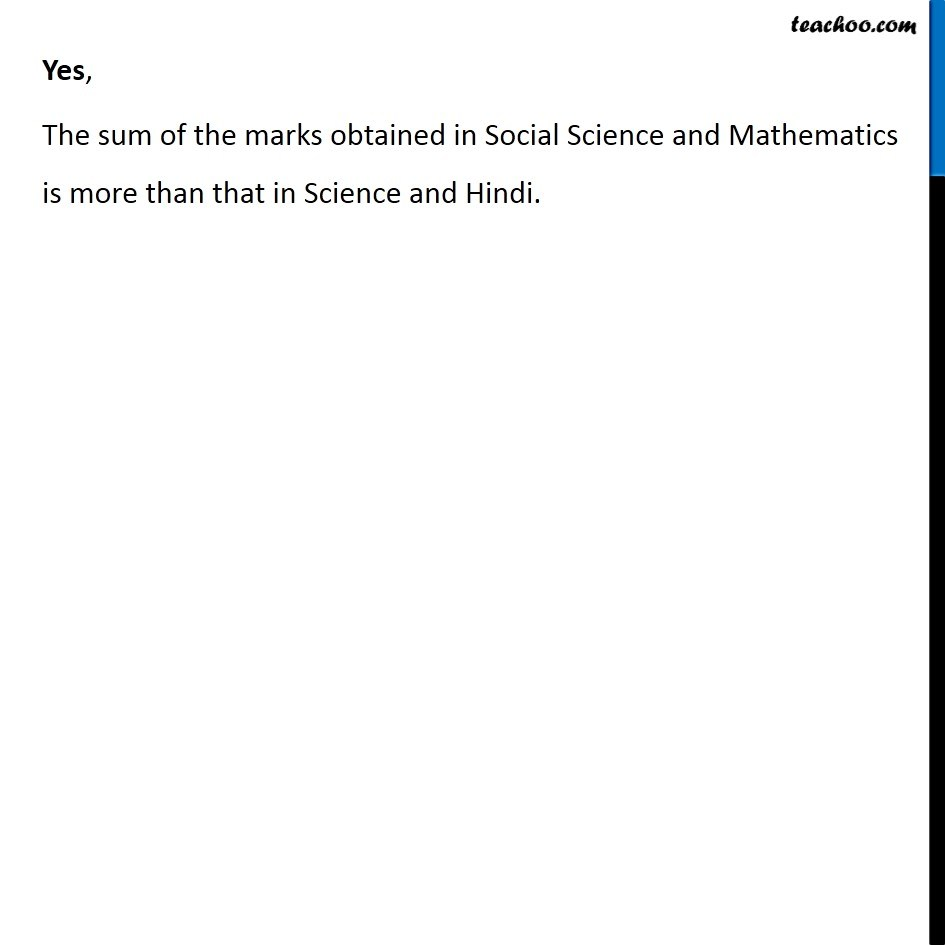Learn in your speed, with individual attention - Teachoo Maths 1-on-1 Class

### Transcript

Ex 4.1, 4 The adjoining pie chart gives the marks scored in an examination by a student in Hindi, English, Mathematics, Social Science and Science. If the total marks obtained by the students were 540, answer the following questions. We need to first find the marks obtained by the students in each subject To find the fraction, we divide angle by 360° because 360° is the central angle of circle.Ex 4.1, 4 (i) In which subject did the student score 105 marks? (Hint: for 540 marks, the central angle = 360°. So, for 105 marks, what is the central angle?) From the table, ∴ The student scored 105 marks in Hindi. Ex 4.1, 4 (ii) How many more marks were obtained by the student in Mathematics than in Hindi? Marks scored in Mathematics = 135 Marks scored in Hindi = 105 Difference in Marks = 135 − 105 = 30 ∴ Students obtained 30 more marks in Mathematics that in Hindi. Ex 4.1, 4 (iii) Examine whether the sum of the marks obtained in Social Science and Mathematics is more than that in Science and Hindi. (Hint: Just study the central angles). Marks in Social Science & Mathematics Marks obtained in Social Science = 97.5 Marks obtained in Mathematics = 135 Sum = 97.5 + 135 = 232.5 Marks in Science & Hindi Marks obtained in Science = 120 Marks obtained in Hindi = 105 Sum = 120 + 105 = 225 Yes, The sum of the marks obtained in Social Science and Mathematics is more than that in Science and Hindi.